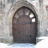Choose Language Hide Translation Bar

## Variance components

Hello all,

I am analyzing data for some quality control task. I have 3 batches from some production. From each one of the 3 batches I have 13 samples. Each sample is a real number between 0 and 1, however I am not sure that 1 is the upper possible limit. Every sample below 0.42 is a failure. I need to find a way to analyze it. Since there are 3 batches, I assume that "batch" is a random effect. I thought that I could analyze it with the variance components, but I find it hard to interpret the results.

Some numerical background: Here are the means and standard deviations of the 3 batches: 0.537 (0.055), 0.656 (0.129) and 0.514 (0.041).

Now, I ran the variance component via the "variability gauge" module (jmp 11), and I got:

ComponentVar component% totalsqrt(var component)
Batch0.005342.60.0729
Within0.007157.40.084
Total0.0121000.111

I am confused with how to interpret this, since the total S.D of all observations together is 0.103, and the sqrt(var component) of the total component is 0.111. What is the difference between these two ?

In addition I got this ANOVA table:

SourceDFSSMS
Batch20.1530.0763
Within360.2570.00716
Total380.4100.01

How does this table add to the variance component one ?

And one last question, is there a way within JMP to show that the minimum is over 0.42, I mean, not just to show the point estimate, but some maybe interval estimation for the minimum ? I just think, maybe if I find a probability of being over 0.42, adjusted for the batch influence.

Thank you !

1 ACCEPTED SOLUTION

Accepted Solutions
Highlightedsusan_walsh1
Staff (Retired)

## Re: Variance components

In the first table, the report is an estimate of variance components based on a linear model where batch is a random effect. The standard deviations cannot be added together to get a total standard deviation. Recall that sqrt(a) + sqrt(b) is not equal to sqrt(a + b). You must add the variances and then take the square root to get the total standard deviation. This may not match the sample standard deviation because it is an estimation based on a linear model, but if the model is a good model it should be close.

The ANOVA table is doing an analysis of variance assuming batch is a fixed effect.susan_walsh1